# Initial¶

In :
!pip -q install "dask[complete]"

In :
!pip -q install "dask-ml[complete]"

In :
!pip -q install --upgrade --ignore-installed numpy pandas scipy sklearn

In :
# https://stackoverflow.com/questions/49853303/how-to-install-pydot-graphviz-on-google-colab?rq=1
!pip -q install graphviz
!apt-get install graphviz -qq
!pip -q install pydot

In :
#!pip -q install bokeh


# Import¶

In :
import numpy as np
import pandas as pd
import graphviz

In :
import matplotlib.pyplot as plt


# 2. Data Types¶

### a) Array:¶

In :
arr = np.random.randint(1, 1000, (1000, 1000))
darr = da.from_array(arr, chunks=(250, 250))
darr

Out:
dask.array<array, shape=(1000, 1000), dtype=int64, chunksize=(250, 250)>
In :
darr.visualize(color="order", size="9,10!")

Out: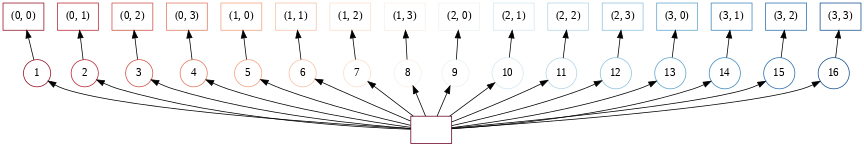In :
darr.chunks, darr.chunksize, darr.npartitions

Out:
(((250, 250, 250, 250), (250, 250, 250, 250)), (250, 250), 16)
In :
res = darr.sum(axis=0)

In :
res.visualize(rankdir="LR", size="3,20!") # Graph of methods we applied
# If we have a graph structure with many independent nodes per level in our implementation, Dask will be able to
# parallelize it and we will get speedup, if our problem is sufficiently large.

Out: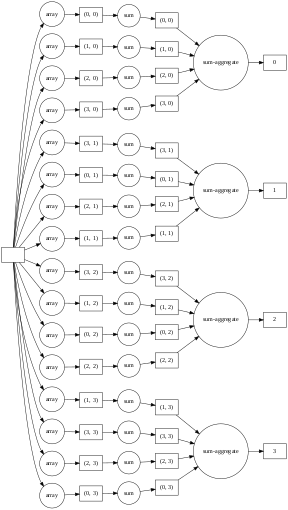In :
res.compute().shape

Out:
(1000,)
In :
def numpy_mean(size=(10, 10)):
arr = np.random.random(size=size)
return arr.mean()

if size > 10000: chunks = (1000, 1000)
else: chunks = (int(size/10), int(size/10))

arr = da.random.random(size=size, chunks=chunks)
y = arr.mean()
return y.compute()

In :
import time

sizes = []
times = []
size = 10
for i in range(5):
dim1 = size ** (i+1)
for j in range(4):
dim2 = size ** (j+1)
if dim1*dim2 in sizes: continue
st = time.time()
en = time.time()
sizes.append(dim1*dim2)
times.append(en-st)
return sizes, times

def numpy_arr_chk():
sizes = []
times = []
size = 10
for i in range(4):
dim1 = size ** (i+1)
for j in range(4):
dim2 = size ** (j+1)
if dim1*dim2 in sizes: continue
st = time.time()
numpy_mean(size=(dim1, dim2))
en = time.time()
sizes.append(dim1*dim2)
times.append(en-st)
return sizes, times

In :
%%time
x1, y1 = numpy_arr_chk()

CPU times: user 37 s, sys: 2.84 s, total: 39.8 s
Wall time: 23.1 s

In :
fig, axs = plt.subplots(1, 3, figsize=(23, 5))
axs.plot(x1[:-1], y1[:-1], "o-", label="Numpy")
axs.set_xlabel("Array elements:")
axs.set_ylabel("Time Taken (sec):")
axs.legend()

axs.plot(x1, y1, "o-", label="Numpy")
axs.set_xlabel("Array elements:")
axs.set_ylabel("Time Taken (sec):")
axs.legend()

axs.plot(x1, y1, "o-", label="Numpy")
axs.set_xlabel("Array elements:")
axs.set_ylabel("Time Taken (sec):")
axs.legend()

Out:
<matplotlib.legend.Legend at 0x7f2dc420db70>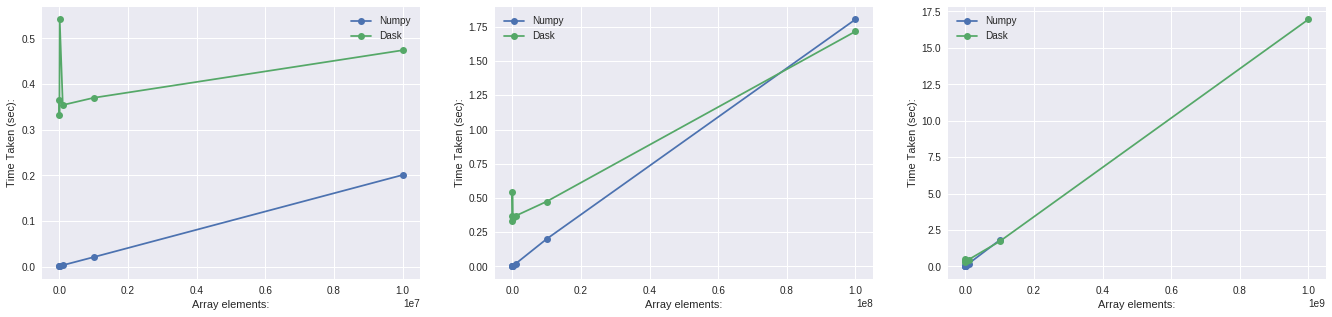In low dimensions, numpy is taking less time than Dask because Dask has to create many processes for the number of workers we set during definition of Client declaration (Fig 1). But as number of array elements increases we see that Dask takes less time than Numpy (Fig 2). Beyond that numpy is not able to compute because it is not able to bring whole array into memory, but Dask is able to by computings many blocks in sequential order. (Fig 3).

### b) DataFrame:¶

In :
import dask.dataframe as dd
import numpy as np
import gc
gc.enable()

In :
arr = np.random.normal(0.0, 1.0, size=(1000000, 10))
df = dd.from_array(arr, chunksize=50000, columns=[f"col-{i+1}" for i in range(10)])
del arr
gc.collect()

Out:
239
In :
df

Out:
col-1 col-2 col-3 col-4 col-5 col-6 col-7 col-8 col-9 col-10
npartitions=20
0 float64 float64 float64 float64 float64 float64 float64 float64 float64 float64
50000 ... ... ... ... ... ... ... ... ... ...
... ... ... ... ... ... ... ... ... ... ...
950000 ... ... ... ... ... ... ... ... ... ...
999999 ... ... ... ... ... ... ... ... ... ...
In :
df.visualize(size="14,16!")

Out: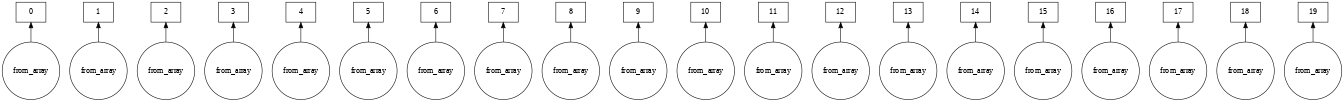In :
df.head() # Not lazy beacuse it doesn't take much computation

Out:
col-1 col-2 col-3 col-4 col-5 col-6 col-7 col-8 col-9 col-10
0 -0.670259 0.130116 1.245027 -1.136380 -0.470103 -0.941553 -1.671139 0.145971 2.293270 0.155823
1 -0.257975 1.908346 -1.550921 -0.457190 -2.577153 -1.934383 -1.645623 -0.591864 1.537130 -2.281100
2 -0.054823 -0.088429 1.380285 0.308579 0.633180 0.193406 0.304017 -1.595323 -0.491884 -0.622066
3 1.232906 1.088529 1.100429 1.195908 1.054931 0.169908 -0.811806 0.355700 1.353377 -0.301842
4 0.664006 -0.243633 -0.429093 -0.274839 2.432883 -0.425719 1.071818 -1.019423 0.236055 1.932841
In :
df.tail()

Out:
col-1 col-2 col-3 col-4 col-5 col-6 col-7 col-8 col-9 col-10
49995 0.247672 0.936369 -1.096501 -0.119473 -0.742709 -1.262157 2.804558 0.123460 -2.267689 -1.635113
49996 1.952103 -0.023558 0.322716 -1.819625 -1.068469 0.395161 -0.089166 0.901499 -1.213241 0.034917
49997 0.217870 0.198978 0.201295 -1.231125 -0.464736 1.505220 1.805124 -0.508538 -0.257614 -1.761602
49998 -0.101036 -0.366966 1.170377 0.325161 -2.288755 1.238234 -1.171790 -1.514991 0.647966 0.392074
49999 1.229795 -1.739559 0.374630 0.652674 0.122733 -1.720617 -1.272090 0.783040 -0.327491 -0.183753
In :
df["col-1"] = (df["col-1"]*10).astype(int)

In :
agg = df.groupby(by=["col-1"]).aggregate(["sum", "std", "max", "min", "mean"])

In :
agg.head(2)

Out:
col-2 col-3 ... col-9 col-10
sum std max min mean sum std max min mean ... sum std max min mean sum std max min mean
col-1
-500 1.048068 NaN 1.048068 1.048068 1.048068 1.437503 NaN 1.437503 1.437503 1.437503 ... -0.720701 NaN -0.720701 -0.720701 -0.720701 -0.544602 NaN -0.544602 -0.544602 -0.544602
-490 0.519506 NaN 0.519506 0.519506 0.519506 1.322778 NaN 1.322778 1.322778 1.322778 ... -0.941289 NaN -0.941289 -0.941289 -0.941289 -0.099877 NaN -0.099877 -0.099877 -0.099877

2 rows × 45 columns

In :
columns = []
for col in agg.columns.levels:
for a in agg.columns.levels:
columns.append(f"{col}.{a}")

agg.columns = columns

Out:
col-10.max col-10.mean col-10.min col-10.std col-10.sum col-2.max col-2.mean col-2.min col-2.std col-2.sum ... col-8.max col-8.mean col-8.min col-8.std col-8.sum col-9.max col-9.mean col-9.min col-9.std col-9.sum
col-1
-500 1.048068 NaN 1.048068 1.048068 1.048068 1.437503 NaN 1.437503 1.437503 1.437503 ... -0.720701 NaN -0.720701 -0.720701 -0.720701 -0.544602 NaN -0.544602 -0.544602 -0.544602
-490 0.519506 NaN 0.519506 0.519506 0.519506 1.322778 NaN 1.322778 1.322778 1.322778 ... -0.941289 NaN -0.941289 -0.941289 -0.941289 -0.099877 NaN -0.099877 -0.099877 -0.099877

2 rows × 45 columns

In :
df_new = df.merge(agg.reset_index(), how="left", on="col-1")

In :
df_new

Out:
col-1 col-2 col-3 col-4 col-5 col-6 col-7 col-8 col-9 col-10 col-10.max col-10.mean col-10.min col-10.std col-10.sum col-2.max col-2.mean col-2.min col-2.std col-2.sum col-3.max col-3.mean col-3.min col-3.std col-3.sum col-4.max col-4.mean col-4.min col-4.std col-4.sum col-5.max col-5.mean col-5.min col-5.std col-5.sum col-6.max col-6.mean col-6.min col-6.std col-6.sum col-7.max col-7.mean col-7.min col-7.std col-7.sum col-8.max col-8.mean col-8.min col-8.std col-8.sum col-9.max col-9.mean col-9.min col-9.std col-9.sum
npartitions=20
int64 float64 float64 float64 float64 float64 float64 float64 float64 float64 float64 float64 float64 float64 float64 float64 float64 float64 float64 float64 float64 float64 float64 float64 float64 float64 float64 float64 float64 float64 float64 float64 float64 float64 float64 float64 float64 float64 float64 float64 float64 float64 float64 float64 float64 float64 float64 float64 float64 float64 float64 float64 float64 float64 float64
... ... ... ... ... ... ... ... ... ... ... ... ... ... ... ... ... ... ... ... ... ... ... ... ... ... ... ... ... ... ... ... ... ... ... ... ... ... ... ... ... ... ... ... ... ... ... ... ... ... ... ... ... ... ...
... ... ... ... ... ... ... ... ... ... ... ... ... ... ... ... ... ... ... ... ... ... ... ... ... ... ... ... ... ... ... ... ... ... ... ... ... ... ... ... ... ... ... ... ... ... ... ... ... ... ... ... ... ... ... ...
... ... ... ... ... ... ... ... ... ... ... ... ... ... ... ... ... ... ... ... ... ... ... ... ... ... ... ... ... ... ... ... ... ... ... ... ... ... ... ... ... ... ... ... ... ... ... ... ... ... ... ... ... ... ...
... ... ... ... ... ... ... ... ... ... ... ... ... ... ... ... ... ... ... ... ... ... ... ... ... ... ... ... ... ... ... ... ... ... ... ... ... ... ... ... ... ... ... ... ... ... ... ... ... ... ... ... ... ... ...
In :
df_new.visualize(rankdir="LR", size="20, 15!")

Out: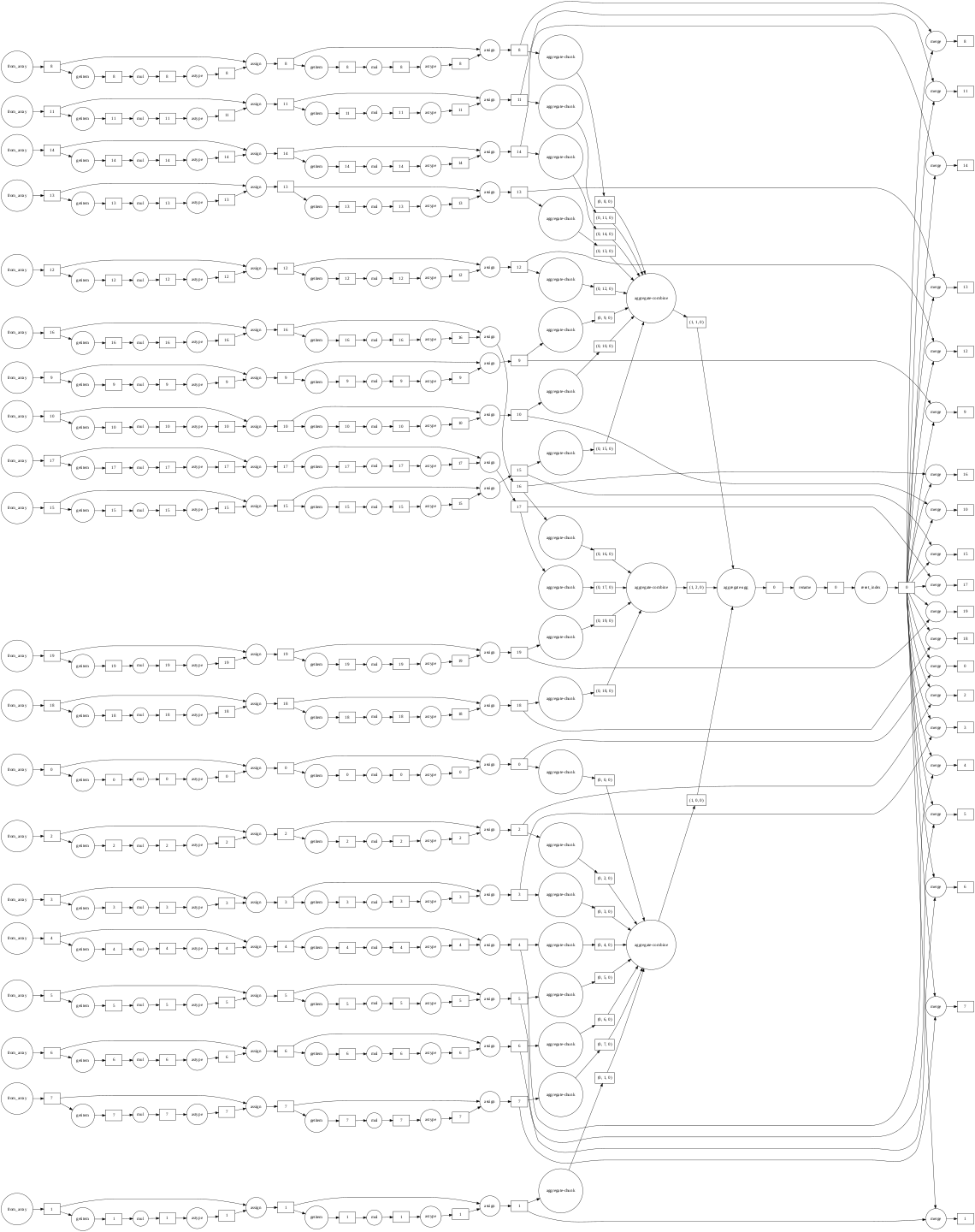In :
df_new.compute().head()

Out:
col-1 col-2 col-3 col-4 col-5 col-6 col-7 col-8 col-9 col-10 ... col-8.max col-8.mean col-8.min col-8.std col-8.sum col-9.max col-9.mean col-9.min col-9.std col-9.sum
0 -60 0.130116 1.245027 -1.136380 -0.470103 -0.941553 -1.671139 0.145971 2.293270 0.155823 ... -436.763233 0.998168 3.957593 -3.943839 -0.013558 -298.565293 0.993525 3.915293 -4.024406 -0.009268
1 -20 1.908346 -1.550921 -0.457190 -2.577153 -1.934383 -1.645623 -0.591864 1.537130 -2.281100 ... 170.245958 1.006248 4.544046 -4.234739 0.004340 158.471773 0.996160 3.838703 -3.834235 0.004040
2 0 -0.088429 1.380285 0.308579 0.633180 0.193406 0.304017 -1.595323 -0.491884 -0.622066 ... -91.181243 1.004919 4.289157 -4.790374 -0.001144 -274.389036 1.000430 3.920591 -4.156023 -0.003441
3 120 1.088529 1.100429 1.195908 1.054931 0.169908 -0.811806 0.355700 1.353377 -0.301842 ... -128.199559 0.993309 3.978109 -3.946863 -0.007043 -15.423090 1.010217 4.344170 -3.792479 -0.000847
4 60 -0.243633 -0.429093 -0.274839 2.432883 -0.425719 1.071818 -1.019423 0.236055 1.932841 ... -116.715633 0.998924 4.284917 -4.321277 -0.003632 -197.993101 1.005309 4.386022 -4.163965 -0.006161

5 rows × 55 columns

In :
df_new.shape.compute(), df_new.shape

Out:
(1000000, 55)

### c) Bag:¶

In :
import dask.bag as db

lst = []
for i in range(5):
lst.append({f"Name.{name}": value for name, value in np.random.randint(1, 10, (5, 2))})
lst.append(np.random.randint(2, 5, (2, 4)))
lst.append(np.random.randint(1, 1000, (1,)))
lst.append([i for i in range(100, 200, 10)])

b = db.from_sequence(lst)
b.take(1)

Out:
({'Name.1': 5, 'Name.2': 4, 'Name.3': 4, 'Name.5': 6, 'Name.8': 4},)
In :
def fil(el):
if type(el)!=dict and type(el)!=list: return True
else: return False

filmap = b.filter(fil).map(lambda x: x**2)

In :
filmap.visualize(size="15,10!")

Out: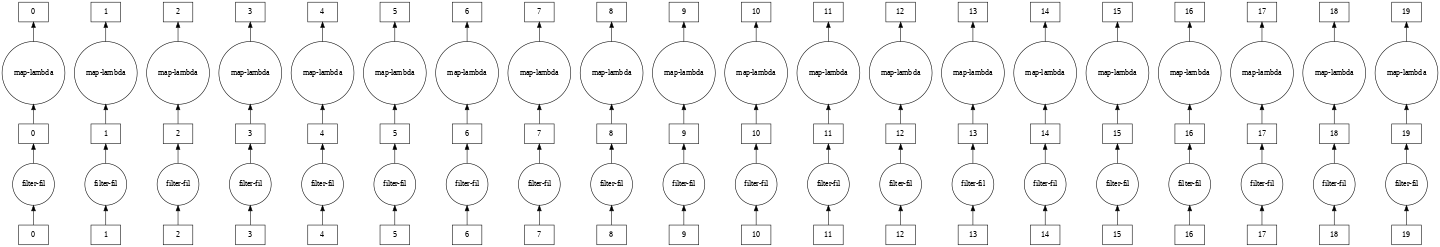In :
filmap.compute()

Out:
[array([[16, 16,  4,  9],
[ 9,  9,  9,  9]]), array(), array([[ 4,  9,  9, 16],
[ 9, 16,  9,  9]]), array(), array([[ 4, 16,  4,  9],
[ 9,  9, 16, 16]]), array(), array([[16,  4, 16,  9],
[16,  9,  4, 16]]), array(), array([[ 4,  9, 16,  9],
[ 4,  9,  9,  9]]), array()]
In :
comp = filmap.flatten().mean()

In :
comp.visualize(size="15, 15!")

Out: